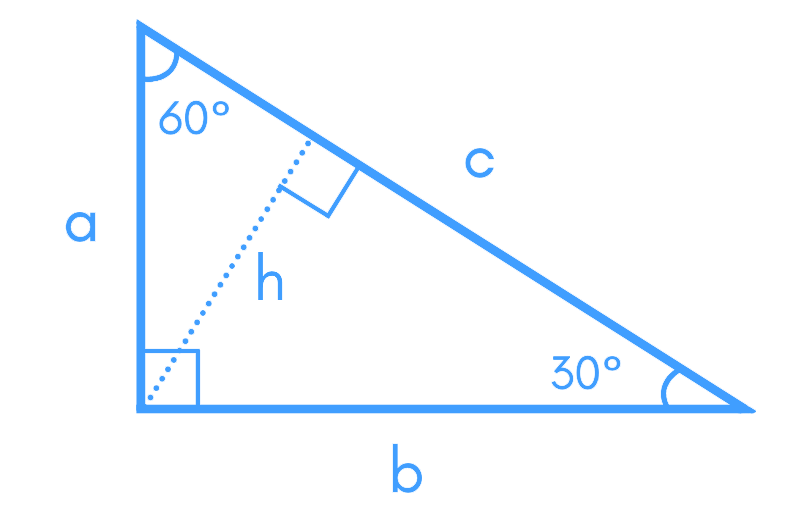# 30 60 90 Triangle Calculator## Table of Content

Feedback

Give the 30 60 90 triangle calculator a try to figure out all the parameters related to any type of triangle. The tool also provides you with step-by-step calculations by using different formulas, thereby ensuring accuracy at its utmost choice.

## What is 30 60 90 Triangle?

A 30 60 90 triangle is a special type in which the measure of three angles are in the ratio 1:2:3, respectively.

As you see, there is an angle of 90 degrees in this geometrical figure, so we can assume it to be a right triangle as well.

## How To Solve a 30 60 90 Triangle?

Well, it’s quite simple! The 30-60-90 triangle calculator considers the following equations to determine all the parameters accurately:### Side Ratios:

The lengths of all three sides in this triangle are not the same. Among these are:

• Longest side of a hypotenuse
• The other two sides as the normal ones that we can also determine with this calculator

### Leg A:

If the measure of the smallest side is a, then we have:

• b = a√3
• c = 2a

### Leg B:

If the measure of the longer side rather than the hypotenuse is given with the perimeter, we have:

• a = b√3/3
• c = 2b√3/3

### Hypotenuse:

If you only have the measure of the hypotenuse, then 30 60 90 triangle formula for the other two sides is as follows:

• a = c/2
• b = c√3/2

### Perimeter:

• P = a × (√3 + 3)

• A = ab/2

## Steps To Use This Calculator!

Want to get instant calculations for the triangle with this tool? Stick to the following guide and get results in seconds!

### What To Do?

• Select the parameter w.r.t which you want to pursue calculations
• Once done, enter the value of the parameter and select its related unit

### What You Get?

You will get the following outputs for the 30 60 90 triangle:

• Height
• Length of the second side
• Area
• Hypotenuse
• Perimeter
• Complete steps involved

### What Are The Ratios In 30 60 90 Triangles?

• For angles: 1 : 2 : 3
• For sides: 1 : √3 : 2

### What Is Special About a 30 60 90 Triangle?

It is the side ratio in 30 60 90 triangles that makes it very unique. Whatever the length of any side you are provided, you can easily calculate the remaining sides by following the aforementioned side ratios.

### Does 9 12 and 15 Make a Right Triangle?

Yes, the combination of side lengths makes a perfect triangle that you can say is a right angles triangle.

### What are the 30 60 90 Triangle Formulas for Trigonometric Ratios?

The trigonometric ratios of the angles in a 30 60 90 triangle can be calculated using the following formulas:

• Sine of 30° = 1/2
• Cosine of 30° = √3/2
• Tangent of 30° = 1/√3
• Sine of 60° = √3/2
• Cosine of 60° = 1/2
• Tangent of 60° = √3

## Citation:

Wikipedia: Special right triangle, Angle-based, Side-based.### Sarah Taylor

I am a professional Chemist/Blogger & Content Writer. I love to research chemistry topics and help everyone learning Organic & Inorganic Chemistry and Biochemistry. I would do anything to spend vacations on a Hill Station.